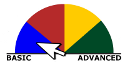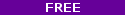# Math

Topics covered in the first two or three semesters of college calculus. Everything from limits to derivatives to integrals to vector calculus. Should understand the topics in the pre-calculus playlist first (the limit videos are in both playlists)Matrices, vectors, vector spaces, transformations, eigenvectors/values. Covers all topics in a first year college linear algebra course. This is an advanced course normally taken by science or engineering majors after taking at least two semesters of calculus (although calculus really isn't a prereq) so don't confuse this with regular high school algebra.Study physics abroad in Europe -- virtually! Learn the basics of physics on location in Italy, the Netherlands and the UK, by answering some of the discipline's major questions from over the last 2000 years. This course is suitable for anyone; a basic understanding of algebra is suggested. This unique class gives you the chance to see the sites where physics history was made and learn some of the subject's most captivating concepts.Projectile motion, mechanics and electricity and magnetism. Solid understanding of algebra and a basic understanding of trigonometry necessary.Introduction to probability. Independent and dependent events. Compound events. Mutual exclusive events. Addition rule for probability.Statistics is about extracting meaning from data. In this class, we will introduce techniques for visualizing relationships in data and systematic techniques for understanding the relationships using mathematics. This course does not require any previous knowledge of statistics. Basic familiarity with algebra such as knowing how to compute the mean, median and mode of a set of numbers will be helpful. This course will cover visualization, probability, regression and other topics that will help you learn the basic methods of understanding data with statistics.How have humans protected their secret messages through history? Apply probability to solve an age old problem.We've always been communicating.... as we moved from signal fires, to alphabets & electricity the problems remained the same.We live in a time of unprecedented access to information...data. Whether researching the best school, job, or relationship, the Internet has thrown open the doors to vast pools of data. Statistics are simply objective and systematic methods for describing and interpreting information so that you may make the most informed decisions about life. It sounds strange to say, but math is not the focus of this class. To do well, however, it is necessary to have a basic understanding of proportions (fractions, decimals, and percentages), negative numbers, basic algebra (solving equations), and exponents and square roots.Videos and exercises on trigonometry. Watch the Khan Academy "Geometry" playlist first if you have trouble understanding the topics covered here.